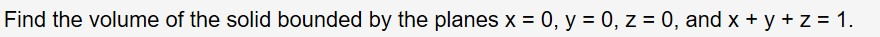# Find the volume of the solid bounded by the planes x 0, yz = 10, z = 0, and x + y

Question
13 viewshelp_outlineImage TranscriptioncloseFind the volume of the solid bounded by the planes x 0, y z = 1 0, z = 0, and x + y fullscreen
check_circle

Step 1

To calculate the volume of the three dimensional region given

Step 2

The required region R (solid) is the bounded volume R shown here. Note that as x travels from 0 to 1, y travels from 0 to 1-x an...

### Want to see the full answer?

See Solution

#### Want to see this answer and more?

Solutions are written by subject experts who are available 24/7. Questions are typically answered within 1 hour.*

See Solution
*Response times may vary by subject and question.
Tagged in

### Integration